Excel 2000 Module 3 Formulas & Functions With Excel, it's easy to perform common calculations. In addition to adding, subtracting, multiplying, and dividing, you can calculate the total and compute the average of a set of values.   Click Practice M2Ex Click Rename to Click Cell A7 Type Total   Formula Concepts A formula is the written expression of a calculation to be performed by Excel. When you enter a formula into a cell, the formula is stored internally while the calculated result appears in the cell. Excel Mathematical Operators (.......) any computation in the bracket; ^ exponential such as 3^2 is 3²; * multiplication such as 3*2; / division such as 3/2; + addition such as 3+2; - subtraction such as 3-2.     Operators and Priority Rules Calculation in Excel follows the Priority Rules in the Operators ( ); ^ ; * or / ; + or - Priority 1 : ( ) Priority 2 : ^ Priority 3 : * or / first-come-first-serve Priority 4 : + or - first-come-first-serve e.g. =1+2*3+(2*3/3) Step 1 (Priority 1): 2*3 first then divided by 3 Step 2 : 2*3 + Step 1 sub total Step 3 : 1 + Step 2 sub total Creating Formulas Creating a formula is similar to entering text and numbers in cells. To begin, you select the cell in which you want the formula to appear. You can use one of two methods to create the formula. In the first method, you type the formula, including cell addresses, constant values, and mathematical operators, directly into the cell. To mark the entry as a formula, you start by typing an equal = sign. Click Cell B8 Type at the Formula bar B5+B6 In the second method, you start by clicking an equal = sign ( button at the Formula bar), then paste the references for a cell or range of cells in the Formula bar. You then complete the formula by typing any operators, constant values, or parentheses. When Excel is in Edit mode, three buttons appear to the left of the Formula bar : , , and .Click Cell C8 Click Cell C5 Type + Click Cell C6 Click on the Formula bar Copying Formulas When you change your mind about the placement of the Formula of a cell, you can change the way you've placed data in your worksheet. Copy & Paste, Cut & Paste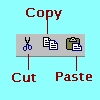Alternatively Use Clipboard Menu, Point and click : click Clipboard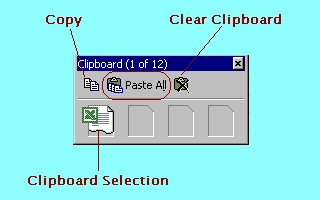Alternatively Use Fill Handle When you select a cell with a formula and drag the Fill handle, Excel changes the cell references in the formula to match those of the column or row to which it has been copied.Select Cell C8, drag Fill handle to D8 Editing Formulas Editing a formula that you have already created is easy and similar to editing the contents of any other cell. Double-click the cell, type your changes directly in the cell, and When Excel is in Edit mode, three buttons appear to the left of the Formula bar : , , and .Click the cell, click in the Formula bar, type your changes, click button on the Formula bar. Click the cell, click Formula button, type your changes in the Formula bar, click button on the Formula bar. Using Absolute and Relative Cell References An absolute reference refers to the address of a specific cell. A relative reference refers to a cell that is a specific rows and columns from the cell that contains the reference. Click Rename to Practice M3Ex Click Cell C4 Create the Formula You will notice how the relative cell referencing error will happen when you copy or use Fill handles on the formulas. Do you notice how the absolute cell referencing for column B does when you copy formulas across. Use key to examine the formula Press to quit To correct the error, we edit the formula. Example : B1 (Column B Row 1) \$B\$1 absolute reference Use key to examine the formula Press to quit No error if we copy formula across or downwards Example : B4 (Column B Row 4) \$B4 absolute reference to Column B, but relative reference to Row 4 Use key to examine the formula Press to quit No error if we copy formula across Example : C3 (Column C Row 3) C\$3 relative reference to Column C, but absolute reference to Row 3 Use key to examine the formula Press to quit No error if we copy formula downwards Using the AutoSum or the SUM Function A function is a predefined formula that performs a common or complex calculation. A function consists of two components : (1) function name; (2) argument list enclosed in ( ). Depending on the function, an argument can be a constant value, a singe-cell reference, a range of cells, a range name, or even another function. When a function contains multiple arguments, the arguments are separated by commas. Click Rename to Use the AutoSum Example at M3: Page 6 Example : =SUM(B4:B6) Click Cell B7 Click button The range B4:B6 is highlighted Click at the Formula bar Use Fill handles at Cell B7 to drag the formula to E7. Using Date Functions Excel's date and time functions allow you to use dates and times in formulas using the functions of DATE ,TIME ,NOW and TODAY. Click Cell A10 Type Before is: Click Cell B10 Click button at the Formula bar Click button Click The Formula Palette appears.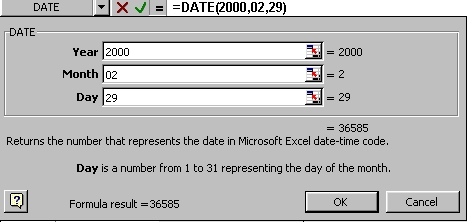Type box 2000 Type box 2 Type box 29 Click Cell A11 Type Today is: Click Cell B11 Click button at the Formula bar Click button Click The Formula Palette appears.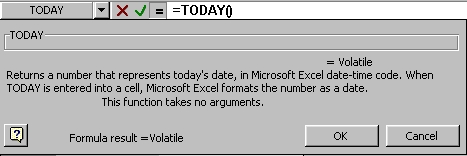Click Using the Formula Paste Functions The Formula Paste Functions offers a third option of entering Formula. The Formula Palette lists each function and its arguments, a description of each function and its arguments, and the calculated result of each function and the overall formula. Click Rename to Click Cell C11 Click button at the Formula bar Click button Click The Formula Palette appears. The Sum function total cells C1:C10 Click Click Cell C12 Click button at the Formula bar Click button Click The Formula Palette appears.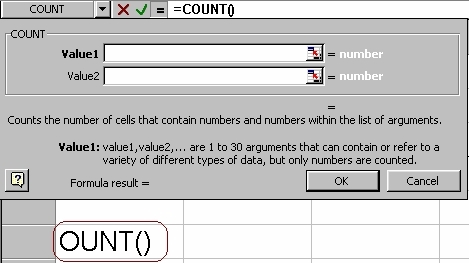Click Select Cells C1:C10 The COUNT function counts cells C1:C10 Click Click Cell C13 or C14 or C15 respectively Click button at the Formula bar Click or or The Formula Palette appears.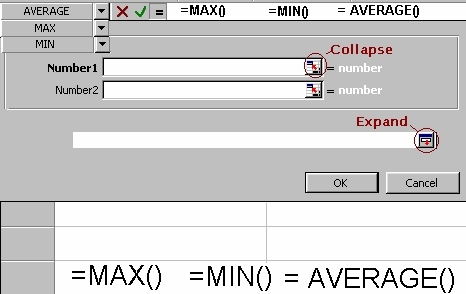Click button at Number 1 box Select C1:C10 Click Auto Calculate On the Status bar at the bottom of the screen, by position the mouse cursor with a right-mouse click, other function commands like Average, Count, Max, Min and Sum appear. Using the COUNTIF (Range, Criteria) Using the COUNTIF function to count the number of cells within a range which meets the given criteria. Click Cell B17 Click button at the Formula bar Click button Click The Formula Palette appears.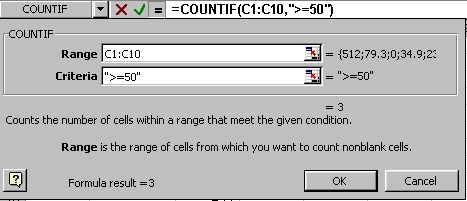Click button at Number 1 box Select C1:C10 Click at Number 2 box Type ">=50" Click Using the IF Function Using the IF function creates a conditional formula. The result of a conditional formula is determined by the state of a specific condition or the answer to a logical question. The IF funcion requires the following syntax: IF(Logical_test, Value_if_true, Value_if_false) Logical test - expression to be evaluated as true or false Value_if_true - value returned if the logical_test expression is true Value_if_false - value returned if the logical_test expression is false Click Rename to Use the IF Example at M3: Page 12 We will determine the PASSED or FAILED grading for the students. Click Cell C2 Click button Click SelectClick Type B2<50 at the Logical Test box Type "FAIL" at the Value_if_true box Type True at the Value_if_false boxClick Using the VLOOKUP Function Using the function to search for a value in the leftmost column of a table, and then returns a value in the same row from a column you specify in the table. =VLOOKUP(LOOKUP VALUE, COMPARE ARRAY, COLUMN INDEX) Click Rename to Use the VLOOKUP Example at M3: Page 14 Click Cell C7 Click button Select Select B7 at the Lookup_value box Select B1:C4 at the Table-array box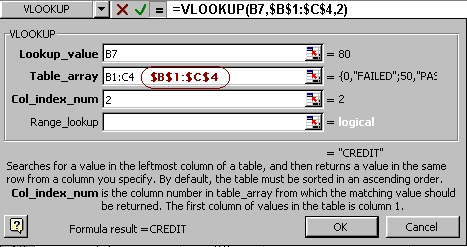Edit \$B\$1:\$C\$4 Type 2 at the Col_index_num box ClickUsing the HLOOKUP Function Using the function to search for a value in the top row of a table or an array of values, and then returns a value in the same column from a row you specify in the table. =HLOOKUP(LOOKUP VALUE, COMPARE ARRAY, ROW INDEX) Click Rename to Use the HLOOKUP Example at M3: Page 15 Click Cell C7 Click button Select Select B5 at the Lookup_value box Select B1:E2 at the Table-array box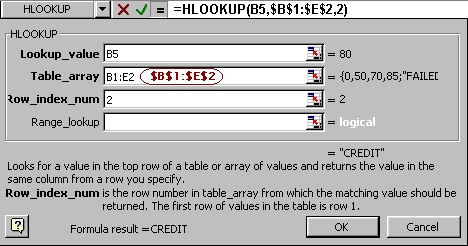Edit \$B\$1:\$E\$2 Type 2 at the Row_index_num box Click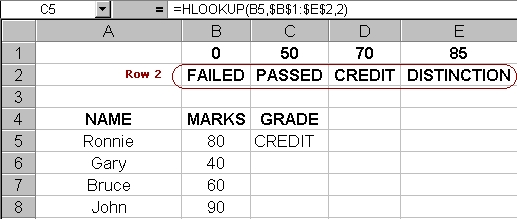Click Click     Practice Exercises   Click My Second Excel2000 at folder Practice Exercise 1 : M3: Page 17 Click Rename to Practice Exercise 2 : M3: Page 18 Click Rename to     Practice Exercise 3 : M3: Page 19 Click Rename to   Practice Exercise 4 : M3: Page 20 Click Rename to     Click Click   Click     Practice Project 1 Practice Excel 2000 Project1   Click Click   ClickEdwin Koh : We completed on the New Knowledge and Skills in Excel 2000 Module 3.

Next Modules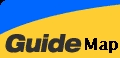Previous Modules